# A hexagonal plate i.s acted upon by the force P and the couple shown. Determine the magnitude and the direction of the smallest force P for which this system can be replaced with a single force at E.

Question-AnswerCategory: Engineering MechanicsA hexagonal plate i.s acted upon by the force P and the couple shown. Determine the magnitude and the direction of the smallest force P for which this system can be replaced with a single force at E.

A hexagonal plate i.s acted upon by the force P and the couple shown. Determine the magnitude and the direction of the smallest force P for which this system can be replaced with a single force at E.Step: 1

Draw the schematic of the hexagonal plate.Step: 2

Identify that the triangle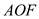is an equilateral triangle. Hence, the distance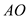is equals to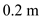.

Step: 3

Determine the net resultant force.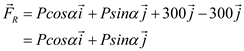…… (1)
Calculate the distanceSubstitute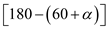for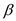, andfor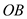.Determine the magnitude of the net moment about point.…… (2)

Step: 4

Represent the net resultant force, and the net moment at pointon the figure.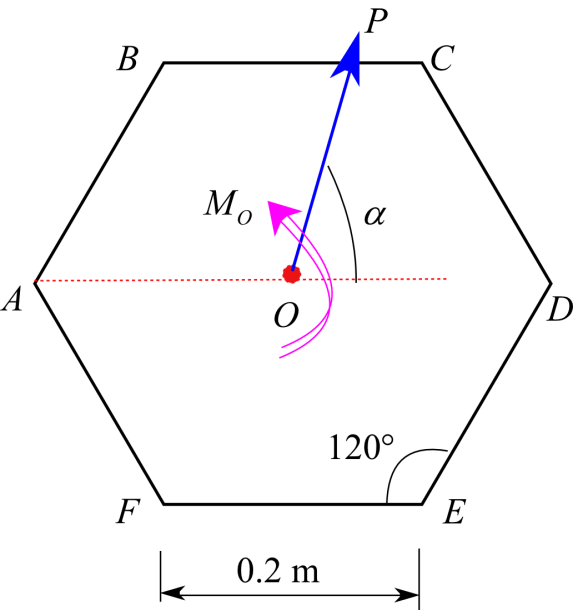Fig. 2
Now let us consider that the moment,and force,may be replaced by force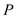making an angle ofwith the horizontal axis at pointas shown below.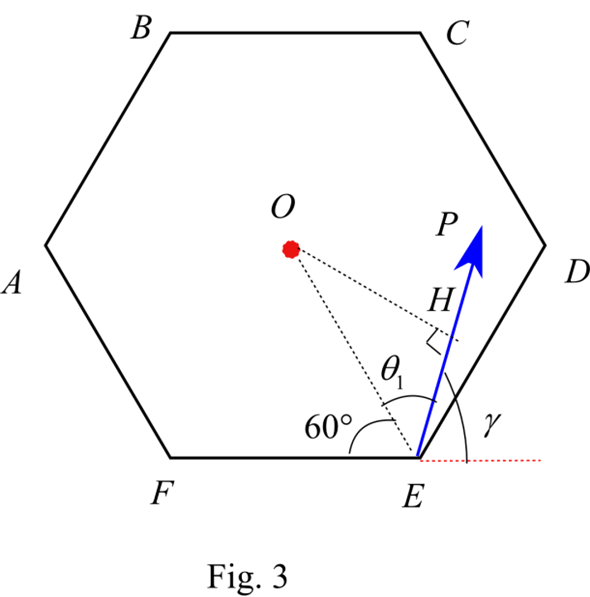Write the vector notation of force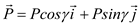…… (3)
Calculate the distance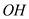Determine the magnitude of the net moment about point(see fig. 3)…… (4)

Step: 5

Equate the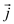components of equations (1) and (3).Therefore, the magnitudes of the anglesand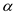are equal.
Equate the equations (2) and (4).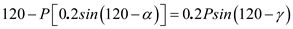Substitutefor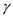.…… (5)

Step: 6

Observe the equation (5).
The magnitude of the forceis minimum when the value ofis maximum.
The maximum value of sine of an angle is 1.
Equate the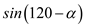to 1.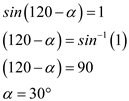Substitute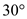forin the equation (5).Therefore, the smallest force,is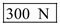making an angle of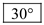with the horizontal.